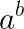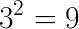# Java Math.pow() Method with Examples

Oct 26, 2016 · 3 mins readThe java.lang.Math class that comes bundled with Java contains various methods for performing basic numeric operations such as the elementary exponential, logarithm, square root, and trigonometric functions.

Math.pow(…) method is part of the Math class. It can be used for calculating the power of a number (base). Let’s look at its method signature:

``````static double Math.pow(double a, double b)
``````

When passed two arguments a and b, it essentially raises a to the power of b and returns the result.Note just like all other methods on the Math class, Math.pow(…) is a `static` method so you can call it directly on the Math class.

Both arguments to Math.pow(…) method are of type `double`. The return type is also `double`. You can cast it to `long` or `int` (be careful because it might overflow.) Let’s raise 3 to the power of 9 using this function and cast the result to integer, that is,``````int result = (int)Math.pow(3, 2) // result = 9
``````

## Code Example

``````import static java.lang.Double.NaN;

public class Main {
public static void main(String[] args) {

System.out.println((long) Math.pow(2, 4)); // 16

System.out.println((long) Math.pow(2, 1)); // 2

System.out.println((long) Math.pow(2, 0)); // 1

// If the second argument is NaN, then the result is NaN.
System.out.println(Math.pow(2, NaN)); // 0

System.out.println(Math.pow(2.5, 3)); // 15.625
}
}
``````

Output

``````16
2
1
NaN
15.625
``````

There you have it. Hope you found this tutorial useful!

### Special cases

Here’s a truncated list of special cases for `Math.pow(...)`, as documented in Javadocs.

• If the second argument is positive or negative zero, then the result is 1.0.
• If the second argument is 1.0, then the result is the same as the first argument.
• If the second argument is NaN, then the result is NaN.
• If the first argument is NaN and the second argument is nonzero, then the result is NaN.
• If
• the absolute value of the first argument is greater than 1 and the second argument is positive infinity, or
• the absolute value of the first argument is less than 1 and the second argument is negative infinity,then the result is positive infinity.
• (truncated)

If you need more detailed information, please see Javadocs. It’s worth noting that the `Math` class in Java contains several other useful methods for arithmetic, logarithms and trignometric operations.

It’s worth noting that the `Math` class in Java contains several useful methods for arithmetic, logarithms and trignometric operations. The following YouTube video below talks about several methods of the `Math` class with examples. It’s a short video and I recommend that you watch it.

#### You May Also Enjoy

• ##### Compare Server-side Rendering vs Client-side Rendering

If you like this post, please share using the buttons above. It will help CodeAhoy grow and add new content. Thank you!